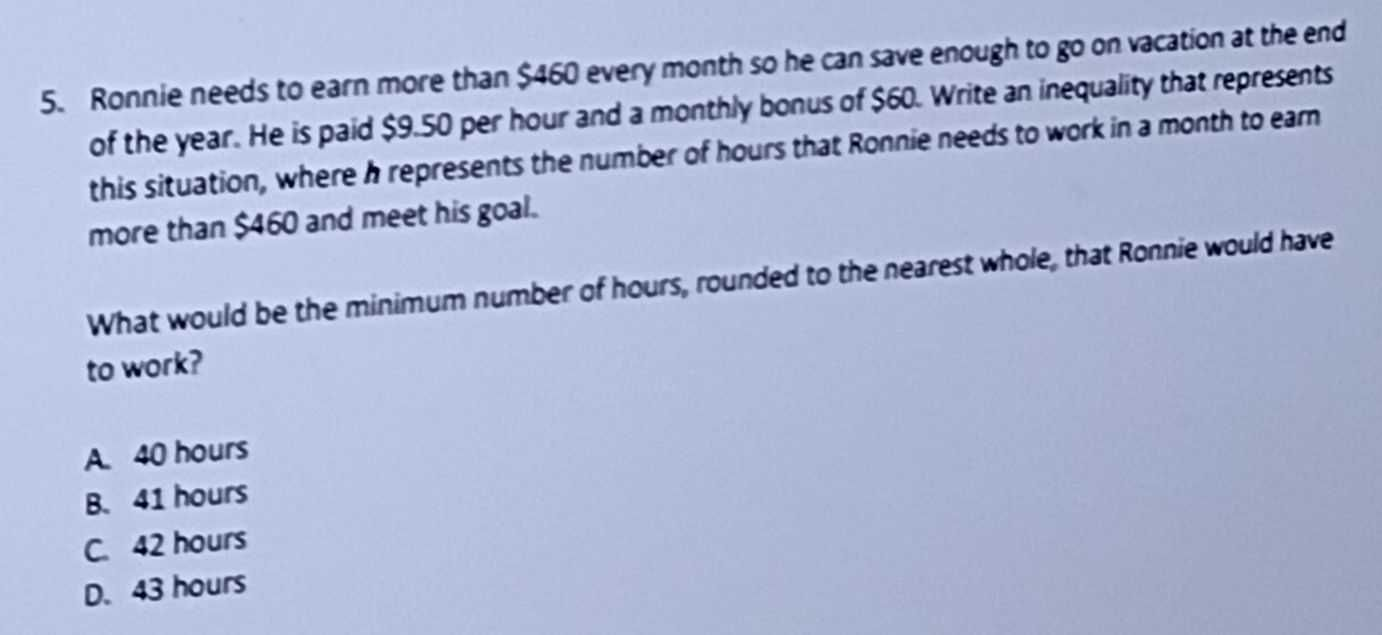### ¿Todavía tienes preguntas de matemáticas?

Pregunte a nuestros tutores expertos
Algebra
Pregunta5. Ronnie needs to earn more than $$\ 460$$ every month so he can save enough to go on vacation at the end of the year. He is paid $$\ 9.50$$ per hour and a monthly bonus of $$\ 60$$ . Write an inequality that represents this situation, where $$h$$ represents the number of hours that Ronnie needs to work in a month to earn more than $$\ 460$$ and meet his goal. What would be the minimum number of hours, rounded to the nearest whole, that Ronnie would have to work?

A. $$40$$ hours B. $$41$$ hours C $$42$$ hours D. $$43$$ hours